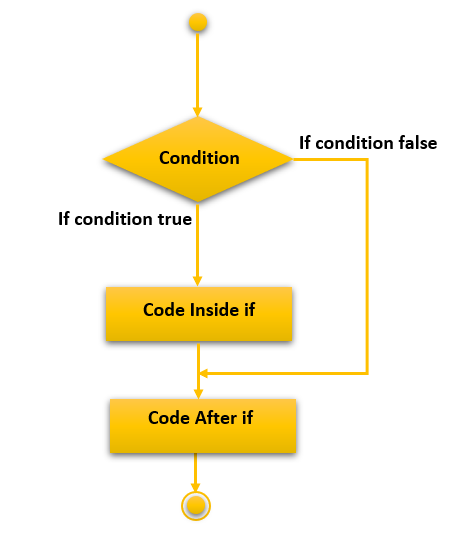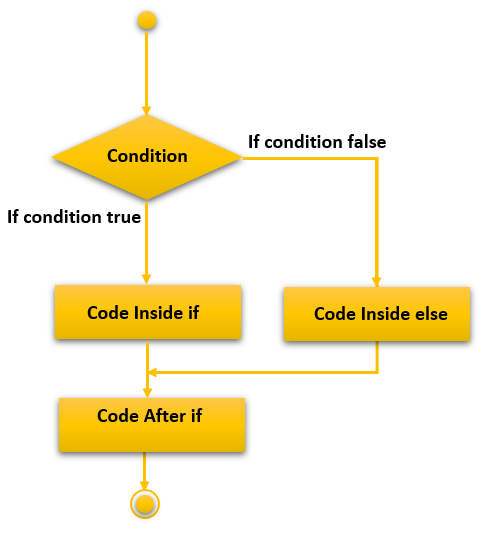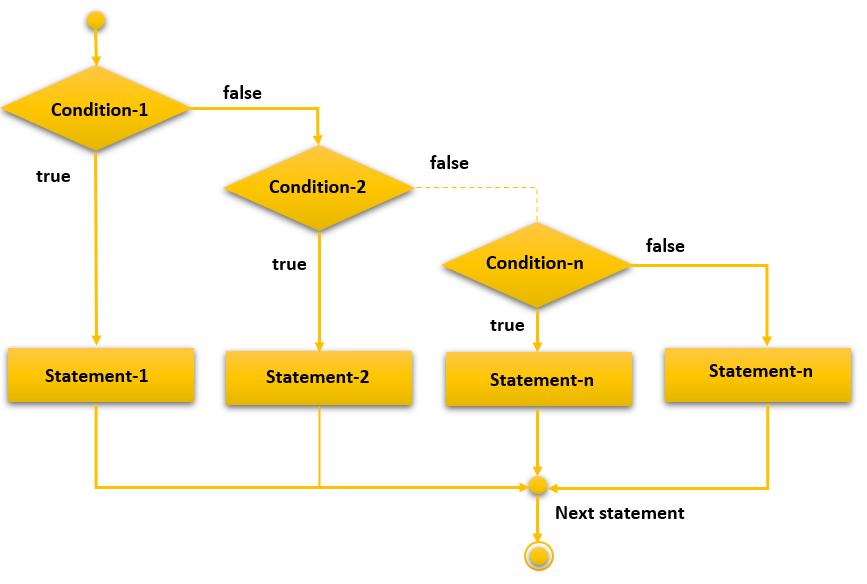# C++ if else statement

In this tutorial, you will learn about the if statement including if…else, if…else…if ladder, and nested if…else in C++ programming language with the help of examples.

In C++, whenever you want to make a choice or a decision in your program and change the execution flow, then you need an `if...else` block. It is a powerful tool that is used to check a particular condition and then execute either the if block or the else block. One should note that only providing an `if` block without an else block will work absolutely fine but vice versa will throw an error. Hence if you only write an if statement with a condition and no else block, the program will run fine but if you only write an else block without any if statement, then you will face a syntax error.

There are the following variants of the if statements available in C++ language.

• If statement
• If-else statement
• Nested if

## C++ if Statement

The if statement is used to perform certain operations only if a given condition is true.

### Syntax

The syntax of the if statement is given below.

```if(test expression){
//code to be executed
}```

### Flowchart of if statement in C++### Example

Let’s check a simple example of `if` statement of C++ language.

```// Program to display a message if it is less than 50
#include <iostream>
using namespace std;
int main() {
int i;
cout << "Enter an integer:";
cin >> i;
// true if number is less than 50
if (i < 50) {
cout <<  "The number is less than 50." << endl;
}
cout << "You have entered:" << i << endl;
return 0;
}```

### Output 1

Enter an integer: 25
The number is less than 50.
You have entered: 25

We enter the number `25`. Hence the body the `if` condition executed and the message `The number is less than 50.` gets printed.

### Output 2

Enter an integer:55
You have entered:55
Now, we have entered a number that is greater than `50`. So the body of the `if` condition not executed.

## C++ if…else Statement

The if…else statement is the extension of the if statement. The if statement performs an operation only if the given condition is correct. Using the if…else statement two operations can be performed i.e. one for the correctness of the given condition another is incorrectness of the given condition. Here, you must remember that the if and else block cannot be executed simultaneously. Either of the ones will be executed.

### Syntax of if…else in C++

The syntax of if…else statement in the  C++ language is as follows.

```if (condition to be checked)
{
// Executes this block if the “if”
// condition is true
}
else
{
// Executes this block if the
// condition is false. No need to provide any condition here.
}```

### Flowchart of if…else statement in C++### Example 1: if statement with an else block

```// C++ program to illustrate if-else statement
#include<iostream>
using namespace std;
int main()
{
int i = 20;
if (i < 15)  // Checking the condition
cout<<"i is smaller than 15";
else
cout<<"i is greater than 15";

return 0;
}```

### Output

i is greater than 15

### Example 2:  if statement without an else block

```// C++ program to illustrate If statement
#include<iostream>
using namespace std;
int main()
{
int i = 10;
if (i > 15)
{
cout<<"10 is less than 15";
}
cout<<"I am Not in if and will always execute";
}```

### Output

I am Not in if and will always execute

In a program you might need to check various scenarios and more than one condition, in that case, you will need an if…else…if ladder structure in C++ where the if the condition is evaluated first and if true, then the rest of the ladder is ignored. If the first condition is false, then it goes over to the immediate next else…if block condition to check if it is true or not and continues to do so until it has checked all of the existing else…if statements or reached the else condition.

### Flowchart of if…else-if ladder statement in C++### Example 1: if…else-if block example

```// C++ program to illustrate if-else-if ladder
#include<iostream>
using namespace std;
int main()
{
int i = 20;
if (i == 10)
cout<<"i is 10";
else if (i == 15)
cout<<"i is 15";
else if (i == 20)
cout<<"i is 20";
else
cout<<"i is not present";
}```

i is 20

### Example 2: if…else-if block without else block.

```// C++ program to illustrate if-else-if ladder
#include<iostream>
using namespace std;
int main()
{
int i = 15;
if (i == 10)
cout<<"i is 10";
else if (i == 15)
cout<<"i is 15";
else if (i == 20)
cout<<"i is 20";
}```

i is 15

## Nested if…else in C++

You can nest one if-else block inside another by simply writing the second if-else block code inside the first if-else block. For example, the following code will explain how a nested if-else works.

```// C++ program to illustrate nested-if statement
#include <iostream>
using namespace std;
int main()
{
int i = 10;
if (i == 10)  // First if statement
{
if (i < 15)
cout<<"i is smaller than 15\n";
// Nested - if statement
// Will only be executed if the first if statement
// is true and then execute the inside block if conditions
if (i < 12)
cout<<"i is smaller than 12 too\n";
else
cout<<"i is greater than 15";
}
return 0;
}```

### Output

i is smaller than 15
i is smaller than 12 too